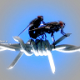### bullets

1.mircea

penal

2.Ashaka

:)))))))))))))))))))))))))))))))))))))))))))))))))))))))))))))))))))))))))))))))))))

3.Dee

Vai ce dragut e 🙂

4.Gabriel Leonard

Pffff…ce stresant. Il omoram dracu` daca aveam un caine care sa faca asa.

5.neXt`Mike

hai ca-l duce capu’ =))